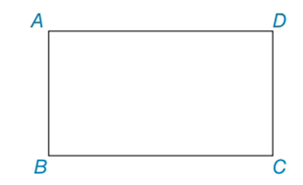Chapter 4.3, Problem 8EElementary Geometry For College St...

7th Edition
Alexander + 2 others
ISBN: 9781337614085

Solutions

Chapter
SectionElementary Geometry For College St...

7th Edition
Alexander + 2 others
ISBN: 9781337614085
Textbook Problem

In Exercises 8 to 10, use the properties of rectangles to solve each problem. Rectangle ABCD is shown in the figure.Exercises 8 - 10 Given: AB = 5 and BC = 12 Find: CD, AD, and AC (not shown)

To determine

To find:

CD, AD, and AC of the given rectangle.

Explanation

The basic property of rectangle:

1) All the properties of a parallelogram apply (the ones that matter here are parallel sides, opposite sides are congruent, and diagonals bisect each other).

2) All angles are right angles by definition.

3) The diagonals are congruent.

Calculation:

Given: AB = 5 and BC = 12

Consider the rectangle.

Now we have to find CD, AD, and AC of the given rectangle.

To find CD and AD:

From the property opposite sides are equal in rectangle.

So, CD = AB = 5

AD = BC = 12

To find AC:

By using Pythagorean Theorem

Still sussing out bartleby?

Check out a sample textbook solution.

See a sample solution

The Solution to Your Study Problems

Bartleby provides explanations to thousands of textbook problems written by our experts, many with advanced degrees!

Get Started

6. Rationalize the denominator: .

Mathematical Applications for the Management, Life, and Social Sciences

In Exercises 1728, use the logarithm identities to obtain the missing quantity.

Finite Mathematics and Applied Calculus (MindTap Course List)

Find the critical numbers of the function. g() = 4 tan

Single Variable Calculus: Early Transcendentals, Volume I